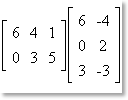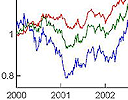Search IntMath
Close

450+ Math Lessons written by Math Professors and Teachers

5 Million+ Students Helped Each Year

1200+ Articles Written by Math Educators and Enthusiasts

Simplifying and Teaching Math for Over 23 Years

# IntMath Newsletter - e Day, funny graphs, risk and matrices

By Murray Bourne, 09 Feb 2011

9 Feb 2011

1. Math tip: Happy "e" Day
2. Funny graphs - from GraphJam
3. Math tip: Multiplying matrices
4. Friday math movie: Hans Rosling's 200 Countries, 200 Years, 4 Minutes
5. Math tip: Determining economic risk - Black Scholes formula
6. Friday math movie: Doodling in math class - binary trees
7. Final thought: Security

Hi everyone. Here's hoping the Year of the Rabbit is a prosperous one for you all - in both learning and living!

(Actually, there is some interesting math associated with rabbits, like Fibonacci's Sequence and differential equations).

## 1. Happy "e" Day

We celebrate "e" day this week, on 7th February (since e = 2.718281828459...). The number "e" is very special in math, in a similar way that "pi" is special.

One of the most amazing equations involving e is Euler's Formula:

eix = cos x + i sin x, where i = √(−1)

This formula makes the connection between the exponential function, trigonometry and the imaginary unit. (See Polar form of a Complex Number.)

In the special case when x = π, we get

eiπ + 1 = 0

This profound formula connects the numbers e, i, π, 1 and 0, with the fundamental operations plus (+) and equals (=).

## 2. Funny graphs - from GraphJam

Suitable for: Everyone.This is fun - our lives in graphs. Funny graphs - from GraphJam

## 3. Multiplying matrices

Suitable for: Everyone. Even if you haven't studied matrices yet, this will give you a good overview of what they are and how to multiply them.This article points to 2 intereactives that show how to multiply matrices. Multiplying matrices

## 4. Friday math movie: Hans Rosling's 200 Countries, 200 Years, 4 Minutes

Suitable for: Everyone.Here's a fascinating animation of wealth trends over the last 200 years. Friday math movie: Hans Rosling's 200 Countries, 200 Years, 4 Minutes

## 5. Determining economic risk - Black Scholes formula

Suitable for: Those who want to see an important application of math. There are many strands of math in this article (logarithms, calculus, statistics, probability).The Black Scholes Model is one of the many formulas used by the mathematical financial wizards to price options. How does it work? Determining economic risk - Black Scholes formula

## 6. Friday math movie: Doodling in math class - binary trees

Suitable for: Everyone.Bored in your math class? Here's some doodles that may make it more interesting, from Di Hart. Friday math movie: Doodling in math class - binary trees

## 7. Final thought – security

This quote comes from the highly successful industrialist, Henry Ford. He made a vast fortune from mass-producing motor vehicles.

If money is your hope for independence you will never have it. The only real security that a man will have in this world is a reserve of knowledge, experience, and ability.

Henry Ford

Until next time, enjoy whatever you learn.

### 4 Comments on “IntMath Newsletter - e Day, funny graphs, risk and matrices”

1. Sihle says:

Thankyou for the newslatter it reallyn helped me,especialy the revision on the numbar 'e' because this week we will be learning to deferentiate hyperbolic functions

Intmath is really amazing,I really from beneath my heart thank you mouray bourne for this amazing creation!!

3. Oxymoron says:

We are easycalculation.com. We serve free online calculators for all kind of business and educational calculations from past some years. We are proud to request you to place our free Rectangular to polar conversion calculator link on your professional site. We hope you have some space for our service. Our Rectangular to polar conversion calculator is very ease to use and also free. You can trust and well-off with our site.

Rectangular to polar conversion Calculator -
https://www.easycalculation.com/area/rectangular-polar-conversion.php

Thank you.

Regards,

4. Murray says:

@Oxymoron: Thanks for the offer, but normally a rectangular to polar conversion involves the origin and one point, not 2 points...

### Comment Preview

HTML: You can use simple tags like <b>, <a href="...">, etc.

To enter math, you can can either:

1. Use simple calculator-like input in the following format (surround your math in backticks, or qq on tablet or phone):
a^2 = sqrt(b^2 + c^2)
(See more on ASCIIMath syntax); or
2. Use simple LaTeX in the following format. Surround your math with $$ and $$.
$$\int g dx = \sqrt{\frac{a}{b}}$$
(This is standard simple LaTeX.)

NOTE: You can mix both types of math entry in your comment.